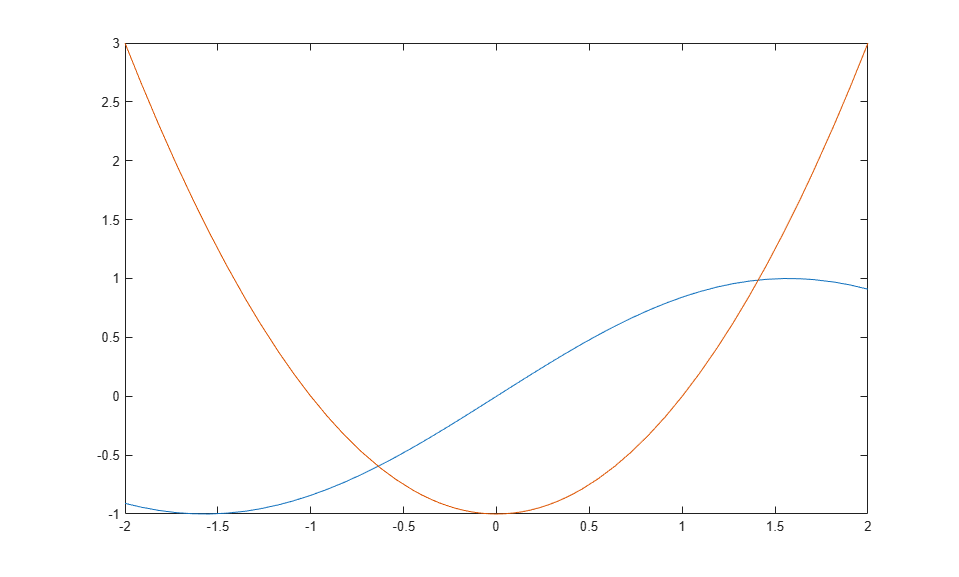# Solving Symbolic Equations

This example shows the basics about solving symbolic equations.

Solve the quadratic equation using the `solve` function.

Solve the quadratic equation without specifying a variable to solve for. The `solve` function chooses `x` to return the solution.

```disp('Solve a quadratic equation without specifying which variable to solve for. The solve function chooses x to return a solution.') disp('>> syms a b c x') disp('>> eqn = a*x^2 + b*x + c == 0') disp('>> S = solve(eqn)') syms a b c x eqn = a*x^2 + b*x + c == 0 S = solve(eqn) ```
```Solve a quadratic equation without specifying which variable to solve for. The solve function chooses x to return a solution. >> syms a b c x >> eqn = a*x^2 + b*x + c == 0 >> S = solve(eqn) eqn = a*x^2 + b*x + c == 0 S = -(b + (b^2 - 4*a*c)^(1/2))/(2*a) -(b - (b^2 - 4*a*c)^(1/2))/(2*a) ```

Specify the variable to solve for and solve the quadratic equation for `a`.

```disp('Solve for the variable a') disp('Sa = solve(eqn,a)') Sa = solve(eqn,a) ```
```Solve for the variable a Sa = solve(eqn,a) Sa = -(c + b*x)/x^2 ```

### Solve Multivariate Equations and Assign Outputs to Structure

When solving for multiple variables, it can be more convenient to store the outputs in a structure array than in separate variables. The `solve` function returns a structure when you specify a single output argument and multiple outputs exist.

Solve a system of equations to return the solutions in a structure array.

```disp('Solve a system of equations to return solutions in a structure array') disp('>> eqns = [2*u + v == 0, u - v == 1];') disp('>> S = solve(eqns,[u v])') syms u v eqns = [2*u + v == 0, u - v == 1]; S = solve(eqns,[u v]) ```
```Solve a system of equations to return solutions in a structure array >> eqns = [2*u + v == 0, u - v == 1]; >> S = solve(eqns,[u v]) S = struct with fields: u: 1/3 v: -2/3 ```

Access the solutions by addressing the elements of the structure.

```disp('Access the solutions within the structure') disp('>> S.u') S.u disp('>> S.v') S.v ```
```Access the solutions within the structure >> S.u ans = 1/3 >> S.v ans = -2/3 ```

Using a structure array allows you to conveniently substitute solutions into other expressions. Use the `subs` function to substitute the solutions `S` into other expressions.

```disp('Use the subs function to substitute the solutions into other expressions') disp('>> e1 = subs(u^2, S)') e1 = subs(u^2,S) disp('>> e2 = subs(3*v + u, S)') e2 = subs(3*v + u,S) ```
```Use the subs function to substitute the solutions into other expressions >> e1 = subs(u^2, S) e1 = 1/9 >> e2 = subs(3*v + u, S) e2 = -5/3 ```

If the `solve` function returns an empty object, then no solutions exist.

```disp('The solve function returns an empty object if no solutions exist') disp('>> solve([3*u+2, 3*u+1],u)') S = solve([3*u+2, 3*u+1],u) ```
```The solve function returns an empty object if no solutions exist >> solve([3*u+2, 3*u+1],u) S = Empty sym: 0-by-1 ```

### Numerically Solve Equations

When the `solve` function cannot symbolically solve an equation, it tries to find a numeric solution using the `vpasolve` function. The `vpasolve` function returns the first solution found.

Try solving the following equation. The `solve` function returns a numeric solution because it cannot find a symbolic solution.

```disp('The following equation returns a numeric solution because the solve function cannot find a symbolic solution') syms x disp('>> eqn = sin(x) == x^2 - 1;') eqn = sin(x) == x^2 - 1; disp('>> solve(eqn,x)') S = solve(eqn,x) ```
```The following equation returns a numeric solution because the solve function cannot find a symbolic solution >> eqn = sin(x) == x^2 - 1; >> solve(eqn,x) Warning: Unable to solve symbolically. Returning a numeric solution using <a href="matlab:web(fullfile(docroot, 'symbolic/vpasolve.html'))">vpasolve</a>. S = -0.63673265080528201088799090383828 ```

Plot the left and the right sides of the equation. Observe that the equation also has a positive solution.

```disp('Plot the left and right sides of the equation to see that the equation also has a positive solution') disp('>> fplot([lhs(eqn) rhs(eqn)], [-2 2])') fplot([lhs(eqn) rhs(eqn)], [-2 2]) ```
```Plot the left and right sides of the equation to see that the equation also has a positive solution >> fplot([lhs(eqn) rhs(eqn)], [-2 2]) ```Find the other solution by directly calling the numeric solver `vpasolve` and specifying the interval.

```disp('Find the other solution by calling the numeric solver vpasolve') disp('>> V = vpasolve (eqn,x,[0,2])') V = vpasolve(eqn,x,[0 2]) ```
```Find the other solution by calling the numeric solver vpasolve >> V = vpasolve (eqn,x,[0,2]) V = 1.4096240040025962492355939705895 ```